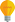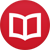# Harmony analysis using numbers (the numeral notation)

by Jakub MarianTip: See my list of theMost Common Mistakes in English. It will teach you how to avoid mis­takes with com­mas, pre­pos­i­tions, ir­reg­u­lar verbs, and much more.

In the previous article, we described a way to think about the notes in the western tonal system as about a line of fifths. This line also provides another natural approach to note naming; we can simply call one of the notes 0 and refer to all other notes by their tonal distance (the distance on the line of fifths) from the reference note. Perhaps the most natural choice for the reference note is D because the C major scale then becomes symmetric around zero, as can bee seen in the following table:

 … D♭ A♭ E♭ B♭ F C G D A E B F♯ C♯ G♯ D♯ … … -7 -6 -5 -4 -3 -2 -1 0 1 2 3 4 5 6 7 …

The convention of denoting notes using Arabic numerals will be called the numeral notation. The easiest way to think about scales in this notation is the following:

A scale that has exactly $n$ sharps (counting flats as negative) consists of notes from $n-3$ to $n+3$.

For example, a scale with 0 sharps (C major/A minor) consists of the notes $-3$, $-2$, $-1$, $0$, $1$, $2$, $3$ (starting with $0-3$ and ending with $0+3$). A scale with 5 sharps (B major/G♯ minor) consists of notes $2$, $3$, $4$, $5$, $6$, $7$, $8$ (starting with $5-3$ and ending with $5+3$). Using the traditional naming convention, these are E, B, F♯, C♯, G♯, D♯, A♯much less obvious, isn’t it?

If you are not interested that much in harmony theory and more in how to use this in practice, you can skip to my article about how to translate chord progressions into any scale easily now.

## Where are the major and minor scales?

It turns out that it is much easier to think merely about the number of sharps the scale we are in has than which major/minor scale it is. Nevertheless, there’s an easy way to convert the two conventions:

The key signature of the $x$ major scale consists of exactly $x+2$ sharps. The key signature of $y$ minor scale consists of exactly $y-1$ sharps.

For example, “$2$ major” (E major) has exactly $2+2 = 4$ sharps, “$0$ major” (D major) has $0+2 = 2$ sharps. Similarly, “$-1$ minor” (G minor) has exactly $-1-1 = -2$ sharps, i.e. 2 flats, “$3$ minor” (B minor) has $3-1 = 2$ sharps. It works the other way round too:

$n$ sharps correspond either to $(n-2)$ major or $(n+1)$ minor.

For example, 3 sharps (i.e. $n = 3$) correspond to $3-2 = 1$ major (A major) or $3+1 = 4$ minor (F♯ minor). In fact, this relation is much more generalevery scale degree corresponds to $n + \text{something}$ where $n$ is the number of sharps (and $\text{something}$ is a number between $-3$ and $3$). More precisely, in the scale with exactly $n$ sharps, we have:

 Note Harmonic function $n-3$ Major Subdominant $n-2$ Major Tonic $n-1$ Major Dominant $n$ Minor Subdominant $n+1$ Minor Tonic $n+2$ Minor Dominant $n+3$ Leading Tone

For example, in the scale with $1$ sharp ($n = 1$), the minor tonic degree is $1+1 = 2 = \text{“E”}$, the major dominant is $1-1 = 0 = \text{“D”}$. The point is that quickly naming any degree of any scale becomes completely trivial (if you can count to 10). Of course, this approach can be used for a simplified harmony analysis and can be easily translated into the traditional notation, but more on that in the next article: [to be continued].

By the way, I have written several educational ebooks. If you get a copy, you can learn new things and support this website at the same time—why don’t you check them out?